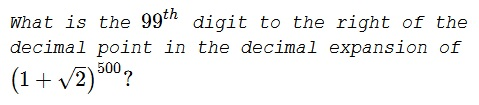# A Remote Decimal Digit

### Problem### Solution

Consider $S=\left(1+\sqrt{2}\right)^{500}+\left(\sqrt{2}-1\right)^{500}.$ $S$ is an integer because the odd powers of $\sqrt{2}$ cancel out. On the other hand, $\left(\sqrt{2}-1\right)^{500}$ is a small number. How small?

$\displaystyle \left(\sqrt{2}-1\right)^{500}\le\left(\frac{1}{2}\right)^{500}\lt\left(\frac{1}{2^7}\right)^{71}\lt\left(\frac{1}{10}\right)^{142}.$

The decimal expansion of $\displaystyle \left(\frac{1}{10}\right)^{142}$ starts with one hundred forthy one $0s$ followed by $1,$ so that the decimal expansion of $\left(\sqrt{2}-1\right)^{500}$ has at least $141$ $0s$ after the decimal point (in fact there are more than $190$ $0s.)$ It follows that $\left(1+\sqrt{2}\right)^{500}$ has as many $9s$ making sure that digit number $99$ is $9.$

### Acknowledgment

This is a problem from P. Winkler's Mathematical Mind-Benders (A K Peters/CRC Press, 2007, p 22).